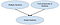# What Are Knewton’s Knowledge Graphs?

## A look into how Knewton represents content to power actionable insights and just-in-time remediation in alta

Imagine you are tutoring a new student who is working on a homework assignment about solving word problems that require two-digit subtraction. The first question on the assignment is:

“Jessica wants to buy a book that costs \$15 and she has \$32 in her wallet. After she buys the book, how much money will she have left?”

Your student looks at the problem and looks back at you, terrified. He has no idea what to do. Clearly he hasn’t yet mastered the ability to solve word problems with two-digit subtraction. But what exactly is the problem? Is he struggling with the word problem itself, or is he missing crucial prerequisite knowledge as well?

Answering this question correctly requires a student to be able to perform the following steps:

1. Translate a word problem about subtraction into a mathematical expression: Question text → 32–15 = ?
2. Solve the two-digit subtraction problem: 32–15
3. Solve one-digit subtraction problems (while performing the two-digit subtraction problem): 12–5, 2–1

From the above breakdown, we can see that this word problem requires a student to know three separate skills, or learning objectives. These learning objectives also happen to build on each other: a student can’t solve a two-digit subtraction problem without first knowing how to solve one-digit subtraction problems, and a student can’t solve word problems that require two-digit subtraction without knowing how to perform two-digit subtraction. We can represent the relationship between these learning objectives in the following way:

In the picture above, we are representing each learning objective as an oval. An arrow pointing from oval A to oval B indicates that a student must know A in order to be successful in B — we describe this relationship by saying that “A is a prerequisite to B”. This simple framework forms the foundation of Knewton’s Knowledge Graphs, a key tool that helps Knewton provide adaptive assignments and analytics in alta.

# Extending the Example

The tiny Knowledge Graph above is a very simple example of how relationships between learning objectives can be represented. In many cases, a given learning objective can have multiple direct prerequisites. A simple example of a learning objective with two prerequisites can be found by looking at the learning objective requiring students to divide fractions. To divide fraction X by fraction Y, you multiply X times the reciprocal of Y. This means that in order to be able to divide fractions, you must already be able to (1) multiply fractions and (2) find reciprocals of fractions.Figure 2: An example learning objective with more than one prerequisite

By connecting each learning objective to its prerequisites, we can create a large Knowledge Graph full of connections between our content. Learning objectives can be connected to each other even if they appear in different assignments, chapters, or courses. Below, we show an example section of a large Knowledge Graph, where different colored learning objectives can be thought of as different assignments, chapters, or course levels.

The notion of representing content relationships in a graph structure is not new, and Knewton’s Knowledge Graphs build on previous work (Novak 1990; McAleese 1999; Doignon and Falmagne 1999; Hwang 2003). Knewton’s Knowledge Graphs are created by trained subject matter experts, who identify the learning objectives in a course, the relationships between these learning objectives, and the pieces of content that teach and assess each learning objective. This direct alignment of each piece of content to learning objectives allows Knewton to precisely diagnose which learning objectives a student has mastered, in addition to providing targeted instructional content when a student is struggling.

# Powering Just-In-Time Remediation

Knewton’s Knowledge Graphs allow us to generate adaptive recommendations based on pedagogical criteria, such as those reviewed by Graesser et al. (2012), including frontier learning, building on prerequisites, and providing remediation. For example, let’s go back to our struggling student. It is possible that our student may not have the prerequisite knowledge necessary to succeed in solving word problems with two-digit subtraction. If he struggles when solving these word problems in an alta adaptive assignment, Knewton’s recommendation engine can diagnose his prerequisite knowledge by using the information contained in the Knowledge Graph and provide just-in-time remediation in the form of targeted instructional content and formative assessment on the prerequisite learning objective(s) that he is struggling with. As the student masters the prerequisites, Knewton can move him forward towards his ultimate goal of learning how to solve word problems with two-digit subtraction.

Since Knewton’s Knowledge Graphs are an abstract representation of relationships between learning objectives, prerequisite relationships can also be defined between learning objectives existing in different books, subject areas, or school years, enabling cross-domain adaptivity. For example, Knewton can recommend remediation on a math prerequisite that’s required for mastering a chemistry learning objective. As we’ll see in a future blog post, Knewton’s Knowledge Graphs are also a key input in the Knewton predictive analytics engine, enabling Knewton to estimate student mastery on each learning objective in a graph.

# Sources

Doignon, J. P. and Falmagne, J. C. (1999). Knowledge Spaces. Springer.

Graesser, A. C., Conley, M. W., and Olney, A. (2012). Intelligent Tutoring Systems. APA Handbook of Educational Psychology. Washington, DC: American Psychological Association.

Hwang, G.-J. (2003). A Conceptual Map Model for Developing Intelligent Tutoring Systems. Computers & Education, 40(3):217–235.

McAleese, R. (1999). Concept Mapping: A Critical Review. Innovations in Education and Training International, 36(4):351–360

Novak, J. D. (1990). Concept Mapping: A Useful Tool for Science Education. Journal of Research in Science Teaching, 27(10):937–949

## Knerd

The Knewton Blog - Stories about technology, product and…

The Knewton Blog - Stories about technology, product and design at Knewton## Knerd

The Knewton Blog - Stories about technology, product and design at Knewton